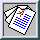Fluid Dynamics Research, Vol. 28 (6) (2001) pp. 397-421
PII: S0169-5983(00)00037-X

## Turbulent penetrative convection with an internal heat source

#### J.R. Chasnov *jeffrey.chasnov@ust.hk and K.L. Tse1

Department of Mathematics, The Hong Kong University of Science and Technology, Clear Water Bay, Kowloon, Hong Kong

Received 14 October 2000; accepted 18 December 2000

### Abstract

An infinite fluid with a vertical cubic temperature profile in the absence of fluid motion is considered as a model for penetrative convection in which a central unstably stratified fluid layer is bounded above and below by stably stratified layers. Turbulence statistics from direct and large eddy numerical simulations for the mean temperature gradient, the velocity and temperature variances and the heat flux are presented for Rayleigh numbers R up to four orders of magnitude above critical. By means of a simplified second-moment closure, analytical scaling laws for the statistics are determined. For high Rayleigh numbers, the mean temperature gradient approaches zero in a central well-mixed layer, a reduced positive (stable) value in upper and lower partially mixed layers, and an unmixed value far above and below. The temperature variance is a factor of R1/3 larger in the partially mixed layers compared to the well-mixed layer; the velocity variance and heat flux scales the same in both layers. Approximation of the three layers by a two layer model yields an estimate for the height of the mixed layer: the height decreases slowly with increasing Rayleigh number and at the highest Rayleigh number simulated is approximately 30% longer than the unstable layer in the absence of fluid motion.

PACS: 47.27.-i; 47.27.Eq

*Corresponding author

1Present address: Department of Mathematics, Arizona State University, Tempe, AZ 85287, USA.

[Full text] (PDF 280.5 Kb)